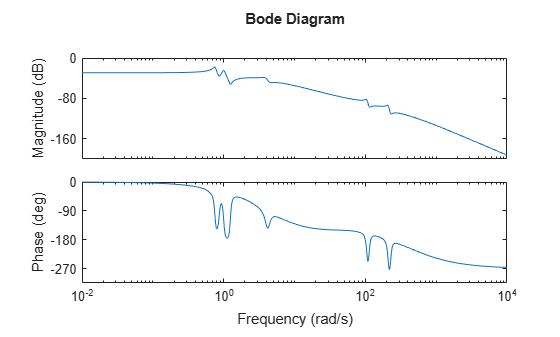# hsvd

Hankel singular values of dynamic system

## Syntax

```hsv = hsvd(sys) hsv = hsvd(sys,opts) [hsv,baldata] = hsvd(___) hsvd(___) ```

## Description

`hsv = hsvd(sys)` computes the Hankel singular values `hsv` of the dynamic system `sys`. In state coordinates that equalize the input-to-state and state-to-output energy transfers, the Hankel singular values measure the contribution of each state to the input/output behavior. Hankel singular values are to model order what singular values are to matrix rank. In particular, small Hankel singular values signal states that can be discarded to simplify the model (see `balred`).

For models with unstable poles, `hsvd` only computes the Hankel singular values of the stable part and entries of `hsv` corresponding to unstable modes are set to `Inf`.

`hsv = hsvd(sys,opts)` computes the Hankel singular values using options that you specify using `hsvdOptions`. Options include offset and tolerance options for computing the stable-unstable decompositions. The options also allow you to limit the HSV computation to energy contributions within particular time and frequency intervals. See `hsvdOptions` for details.

`[hsv,baldata] = hsvd(___)` returns additional data to speed up model order reduction with `balred`. You can use this syntax with any of the previous combinations of input arguments.

`hsvd(___)` displays a Hankel singular values plot.

## Examples

collapse all

Create a system with a stable pole very near to 0, and display the Hankel singular values.

```sys = zpk([1 2],[-1 -2 -3 -10 -1e-7],1); hsv = hsvd(sys)```
```hsv = 5×1 105 × 1.6667 0.0000 0.0000 0.0000 0.0000 ```

Notice the dominant Hankel singular value with magnitude $1{0}^{5}$, which is so much larger that the significant digits of the other modes are not displayed. This value is due to the near-unstable mode at $s=1{0}^{-7}$. Use the `'Offset'` option to treat this mode as unstable.

```opts = hsvdOptions('Offset',1e-7); hsvu = hsvd(sys,opts)```
```hsvu = 5×1 Inf 0.0688 0.0138 0.0024 0.0001 ```

The Hankel singular value of modes that are unstable, or treated as unstable, is returned as `Inf`. Create a Hankel singular-value plot while treating this mode as unstable.

`hsvd(sys,opts)`The unstable mode is shown in red on the plot.

By default, `hsvd` uses a linear scale. To switch the plot to a log scale, right-click on the plot and select Y Scale > Log. For information about programmatically changing properties of HSV plots, see `hsvplot`.

Compute the Hankel singular values of a model with low-frequency and high-frequency dynamics. Focus the calculation on the high-frequency modes.

Load the model and examine its frequency response.

```load modeselect Gms bodeplot(Gms)````Gms` has two sets of resonances, one at relatively low frequency and the other at relatively high frequency. Compute the Hankel singular values of the high-frequency modes, excluding the energy contributions to the low-frequency dynamics. To do so, use `hsvdOptions` to specify a frequency interval above 30 rad/s.

```opts = hsvdOptions('FreqInterval',[30 Inf]); hsvd(Gms,opts)```## Tips

To create a Hankel singular-value plot with more flexibility to programmatically customize the plot, use `hsvplot`.

## Algorithms

The `AbsTol`, `RelTol`, and `Offset` options of `hsvdOptions` are only used for models with unstable or marginally stable dynamics. Because Hankel singular values are only meaningful for stable dynamics, `hsvd` must first split such models into the sum of their stable and unstable parts:

``` G = G_s + G_ns ```

This decomposition can be tricky when the model has modes close to the stability boundary (e.g., a pole at `s=-1e-10`), or clusters of modes on the stability boundary (e.g., double or triple integrators). While `hsvd` is able to overcome these difficulties in most cases, it sometimes produces unexpected results such as

1. Large Hankel singular values for the stable part.

This happens when the stable part `G_s` contains some poles very close to the stability boundary. To force such modes into the unstable group, increase the `'Offset'` option to slightly grow the unstable region.

2. Too many modes are labeled "unstable." For example, you see 5 red bars in the HSV plot when your model had only 2 unstable poles.

The stable/unstable decomposition algorithm has built-in accuracy checks that reject decompositions causing a significant loss of accuracy in the frequency response. Such loss of accuracy arises, e.g., when trying to split a cluster of stable and unstable modes near `s=0`. Because such clusters are numerically equivalent to a multiple pole at `s=0`, it is actually desirable to treat the whole cluster as unstable. In some cases, however, large relative errors in low-gain frequency bands can trip the accuracy checks and lead to a rejection of valid decompositions. Additional modes are then absorbed into the unstable part `G_ns`, unduly increasing its order.

Such issues can be easily corrected by adjusting the `AbsTol` and `RelTol` tolerances. By setting `AbsTol` to a fraction of smallest gain of interest in your model, you tell the algorithm to ignore errors below a certain gain threshold. By increasing `RelTol`, you tell the algorithm to sacrifice some relative model accuracy in exchange for keeping more modes in the stable part `G_s`.

If you use the `TimeIntervals` or `FreqIntervals` options of `hsvdOptions`, then `hsvd` bases the computation of state energy contributions on time-limited or frequency-limited controllability and observability Gramians. For information about calculating time-limited and frequency-limited Gramians, see `gram` and .

## References

 Gawronski, W. and J.N. Juang. “Model Reduction in Limited Time and Frequency Intervals.” International Journal of Systems Science. Vol. 21, Number 2, 1990, pp. 349–376.